• 如果您觉得本站非常有看点，那么赶紧使用Ctrl+D 收藏吧

# 如何使用JavaScript实现前端导入和导出excel文件(H5编辑器实战复盘)

6天前 7次浏览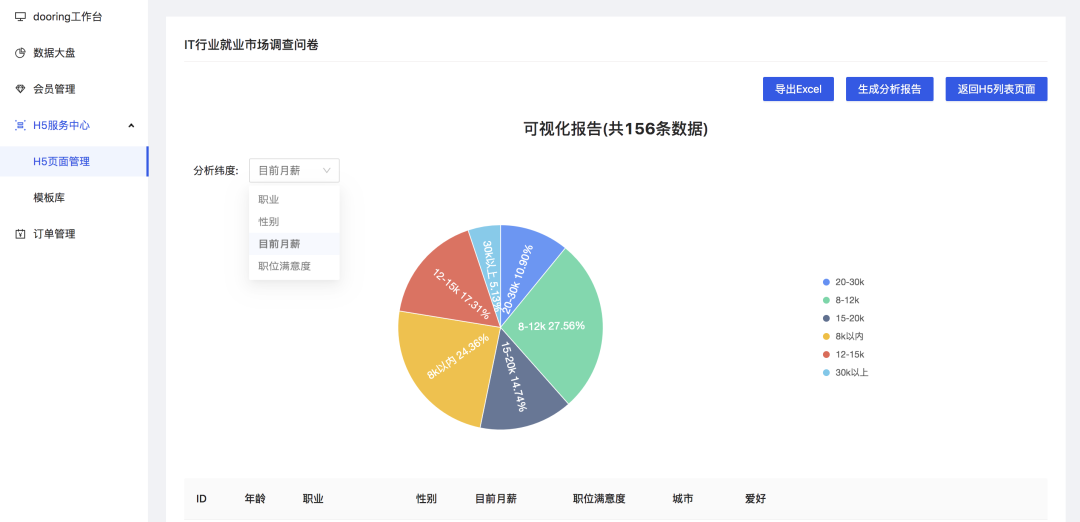## 前言

• 如何使用JavaScript实现前端导入和导出excel文件(H5编辑器实战复盘)
• 前端如何基于table中的数据一键生成多维度数据可视化分析报表
• 如何实现会员管理系统下的权限路由和权限菜单

• 使用JavaScript实现前端导入excel文件并自动生成可编辑的Table组件
• 使用JavaScript实现前端基于Table数据一键导出excel文件
• XLSXjs-export-excel基本使用

## 正文

### 1. 使用JavaScript实现前端导入excel文件并自动生成可编辑的Table组件

#### 1.2 实现一键导入excel文件并生成table表格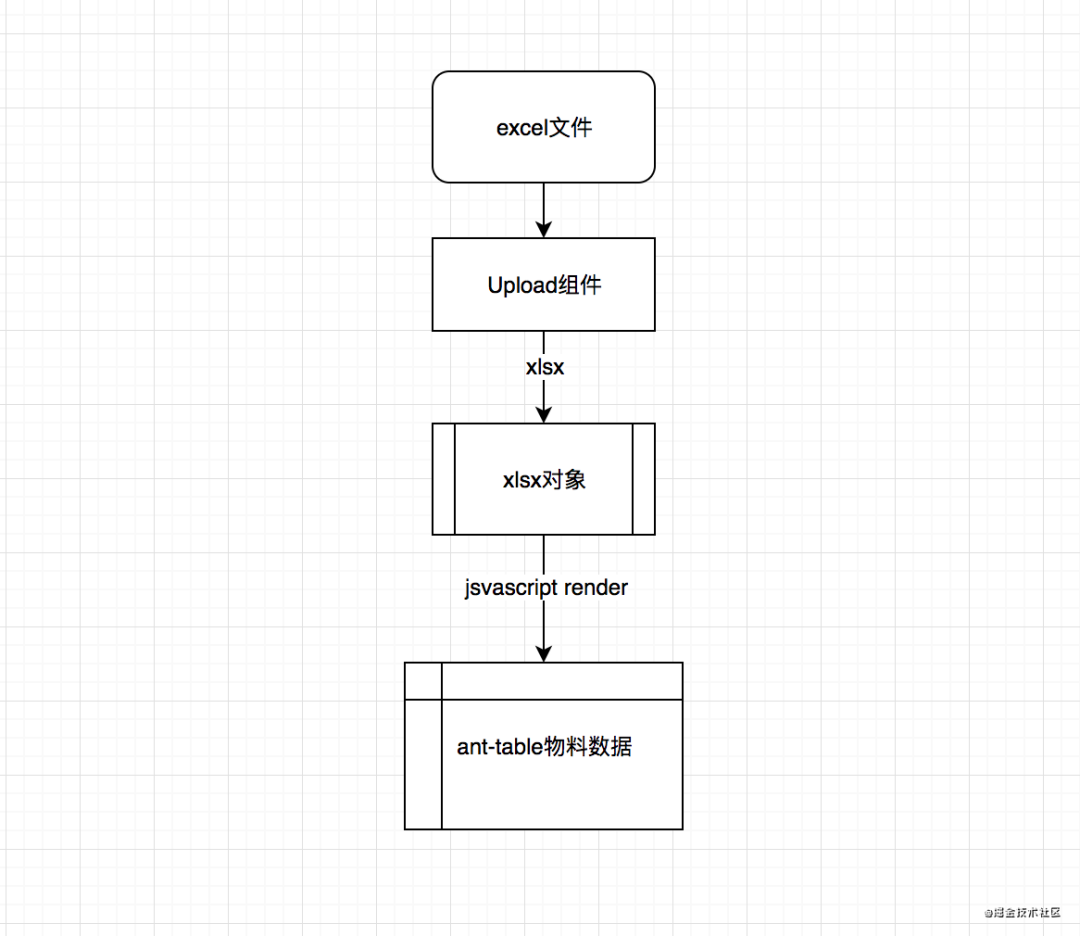``````

// 解析并提取excel数据let reader = new FileReader();reader.onload = function(e) {  let data = e.target.result;  let workbook = XLSX.read(data, {type: 'binary'});  let sheetNames = workbook.SheetNames; // 工作表名称集合  let draftObj = {}  sheetNames.forEach(name => {    // 通过工作表名称来获取指定工作表    let worksheet = workbook.Sheets[name];     for(let key in worksheet) {      // v是读取单元格的原始值      if(key !== '!') {        if(draftObj[key]) {          draftObj[key].push(worksheet[key].v)        }else {          draftObj[key] = [worksheet[key].v]        }      }    }  });  // 生成ant-table支持的数据格式  let sourceData = Object.values(draftObj).map((item,i) => ({ key: i + '', name: item, value: item}))

复制代码

``````

#### 1.3 table表格的编辑功能实现

table表格的编辑功能实现其实也很简单, 我们只需要按照antd的table组件提供的自定义行和单元格的实现方式即可. antd官网上也有实现可编辑表格的实现方案, 如下: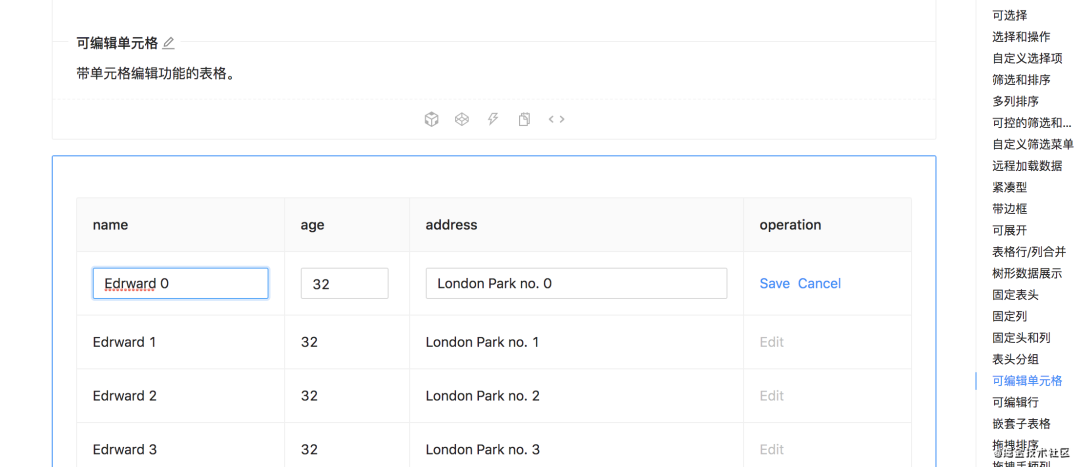#### 1.4 根据编辑的table数据动态生成图表

``````

const data = [  { genre: 'Sports', sold: 275 },  { genre: 'Strategy', sold: 115 },  { genre: 'Action', sold: 120 },  { genre: 'Shooter', sold: 350 },  { genre: 'Other', sold: 150 }];

复制代码

``````

``````

import { Chart } from '@antv/f2';import React, { memo, useEffect, useRef } from 'react';import ChartImg from '@/assets/chart.png';import styles from './index.less';import { IChartConfig } from './schema';interface XChartProps extends IChartConfig {  isTpl: boolean;}const XChart = (props: XChartProps) => {  const { isTpl, data, color, size, paddingTop, title } = props;  const chartRef = useRef(null);  useEffect(() => {    if (!isTpl) {      const chart = new Chart({        el: chartRef.current || undefined,        pixelRatio: window.devicePixelRatio, // 指定分辨率      });      // step 2: 处理数据      const dataX = data.map(item => ({ ...item, value: Number(item.value) }));      // Step 2: 载入数据源      chart.source(dataX);      // Step 3：创建图形语法，绘制柱状图，由 genre 和 sold 两个属性决定图形位置，genre 映射至 x 轴，sold 映射至 y 轴      chart        .interval()        .position('name*value')        .color('name');      // Step 4: 渲染图表      chart.render();    }  }, [data, isTpl]);  return (    <div className={styles.chartWrap}>      <div className={styles.chartTitle} style={{ color, fontSize: size, paddingTop }}>        {title}      </div>      {isTpl ? <img src={ChartImg} alt="dooring chart" /> : <canvas ref={chartRef}></canvas>}    </div>  );};export default memo(XChart);

复制代码

``````

### 2. 使用JavaScript实现前端基于Table数据一键导出excel文件

#### 2.1 一键导出为excel实现效果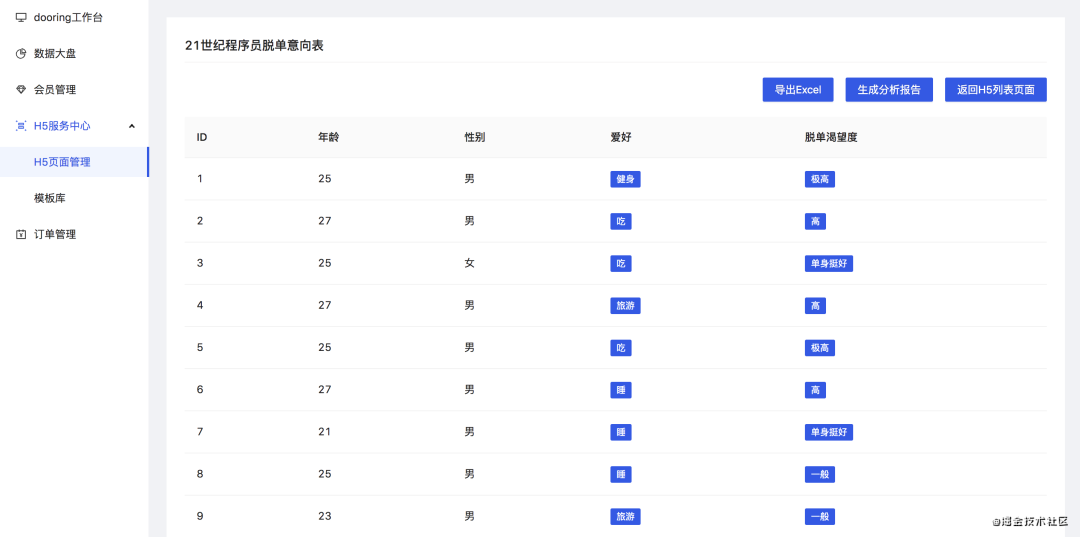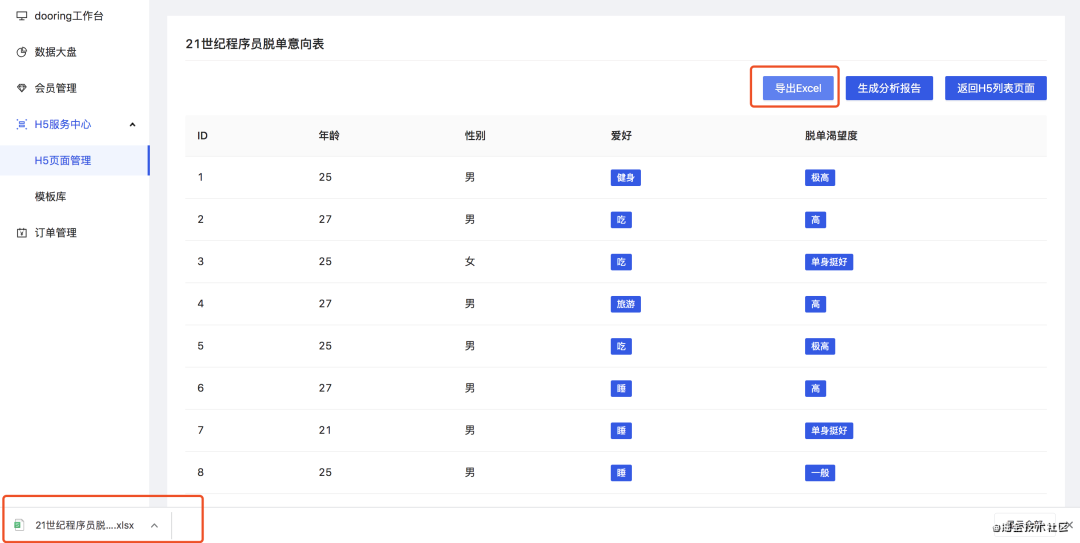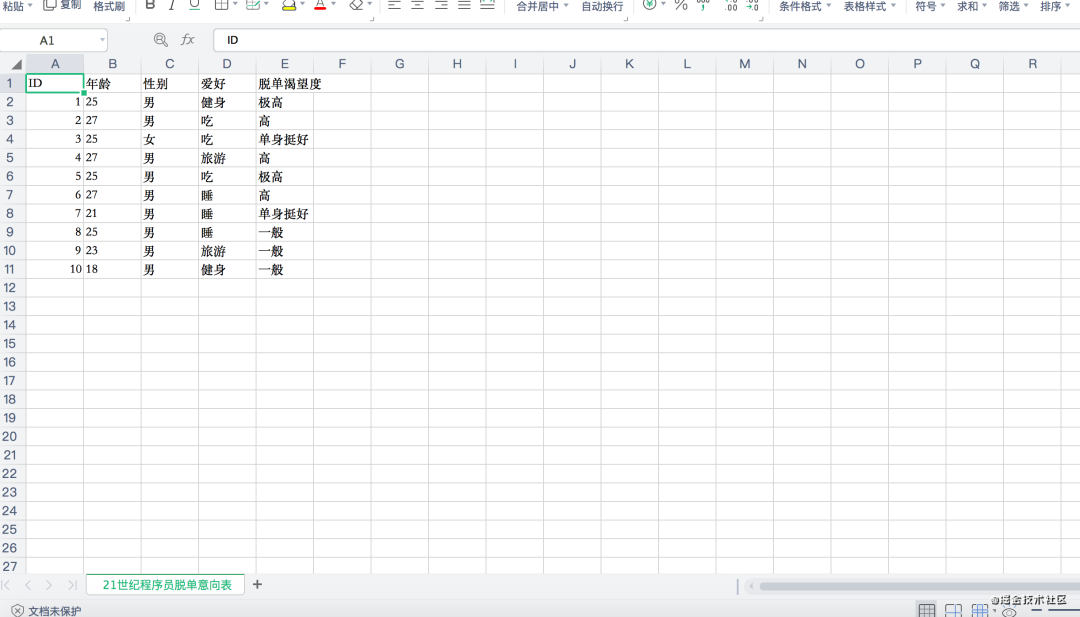以上就是用户基于后台采集到的数据, 一键导出excel文件的流程, 最后一张图是生成的excel文件在office软件中的呈现.

#### 2.2 使用javascript实现一键导出excel文件功能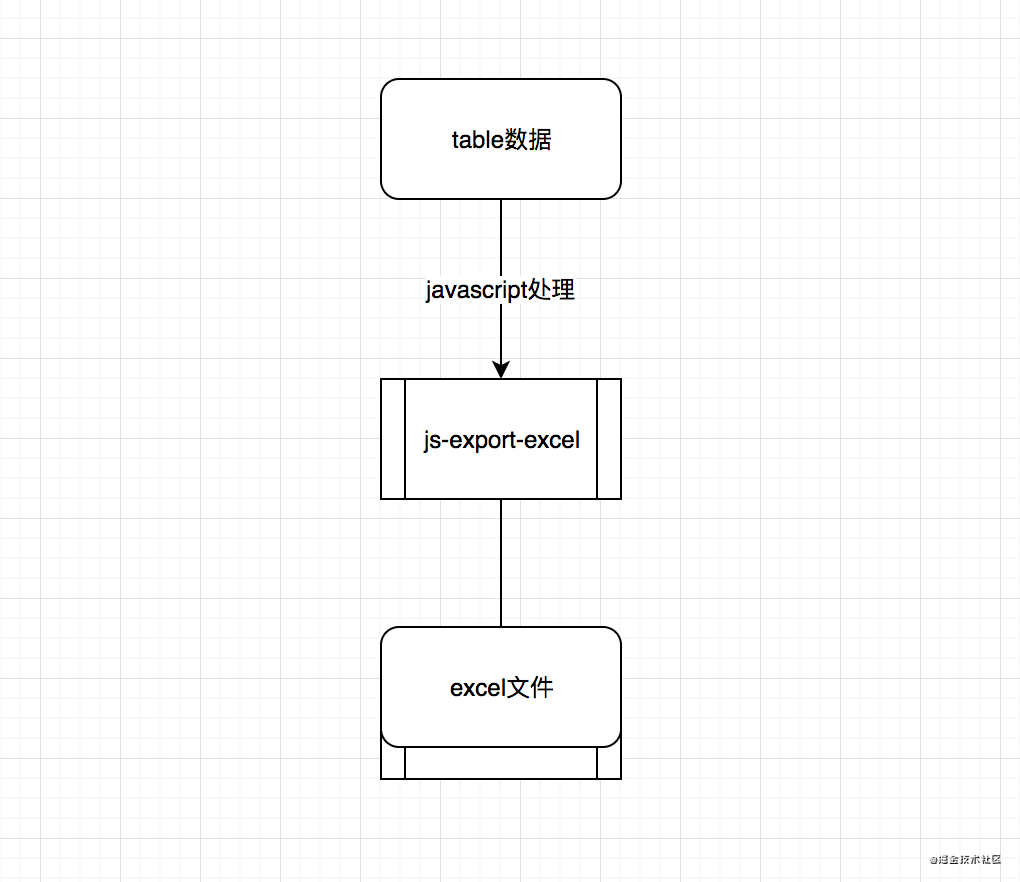• 自定义导出的excel文件名
• 自定义excel的过滤字段
• 自定义excel文件中每列的表头名称

``````

const generateExcel = () => {    let option = {};  //option代表的就是excel文件    let dataTable = [];  //excel文件中的数据内容    let len = list.length;    if (len) {        for(let i=0; i<len; i++) {            let row = list[i];            let obj:any = {};            for(let key in row) {                if(typeof row[key] === 'object') {                    let arr = row[key];                    obj[key] = arr.map((item:any) => (typeof item === 'object' ? item.label : item)).join(',')                }else {                    obj[key] = row[key]                }            }            dataTable.push(obj);  //设置excel中每列所获取的数据源        }    }    let tableKeys = Object.keys(dataTable);    option.fileName = tableName;  //excel文件名称    option.datas = [          {            sheetData: dataTable,  //excel文件中的数据源            sheetName: tableName,  //excel文件中sheet页名称            sheetFilter: tableKeys,  //excel文件中需显示的列数据            sheetHeader: tableKeys,  //excel文件中每列的表头名称          }    ]    let toExcel = new ExportJsonExcel(option);  //生成excel文件    toExcel.saveExcel();  //下载excel文件  }

复制代码

``````

注意, 以上笔者实现的方案对任何table组件都使用, 可直接使用以上代码在大多数场景下使用. 至此, 我们就实现了使用JavaScript实现前端导入和导出excel文件的功能.

## 最后

github🔍：H5在线编辑器H5-Dooring